# MACRO-UNION

The macro-union operator of MAD is proposed in Fortunato et al. (1986). It was subsequently reproposed with the name of fusion. It works on two operands and, if necessary, recomputes the numeric values of the measure. In other words, given two MADs with common schemas, this operator provides a new MAD in output by taking the set-union of the facts described by the two MADs in input, and of the fact-dimension relations. This means that the definition domains of each category attribute of the output MAD are the union of the definition domains of the corresponding category attributes in the input MAD. Partial overlappings can happen between two category attributes with the same primitive definition domain. Null values can appear, with the meaning of "not available measured data" (‘NA’). Operating only on one dimension, it performs the union of the categories and the partial orders. An example of a macro-union operator application is shown in Figures 12 and 13.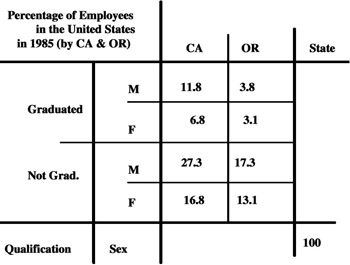Figure 12a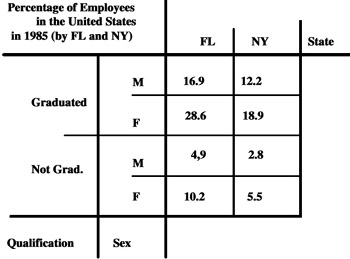Figure 12b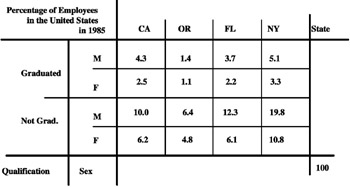Figure 13

It is similar to the union operator, proposed in Gyssens & Lakshamanan (1997), Pedersen & Jensen (1999), and Pedersen, Jensen, & Dyreson (2001), where it is applied only on one dimension, to the set-union operator, proposed in Ozsoyoglu, Ozsoyoglu, & Matos (1987), and to enlargement, proposed in Rafanelli & Ricci (1993).

Obviously, in order to have a MAD in output which makes sense, each pair of category attributes combined together have to belong to the same dimension (generally the same hierarchy and often the same level of this hierarchy).

It is important to note, similarly to the Restriction operator discussed in the following, that also this operator depends on the summary type of its measure. In fact, if its summary type had been "percentage," as in Figure 13, the single values inside the cells of the MAD would have had to be recomputed in order to maintain consistency in the information. For this recomputation we need a predefined distribution function, represented by a table TD, or, often, by an expression which shows the rate which defines either the "weight" by which to compute the data in the MAD in output, or the way to relate this weight to the summary data in the MAD in input.

For example, let T1 be the MAD, shown in Figure 12 (a), which we wish to enlarge, and let T2 be the MAD to unite with T1. If TD is represented by the expression "100 (T1) = 36,7 (Tout)," the resulting MAD in output is shown in Figure 13.

Let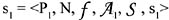be a MAD which describes a given fact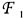of a given phenomenon and which is defined on its MAD schema A1 = {A1j}, and let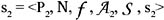be another MAD which describes another fact F2 of the same phenomenon and which is defined on its MAD schema A2 = {A2j}.

The union of s1 and s2 produces a new MAD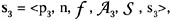where N, f, and S are the same of and s1 and s2,

p3 is the new name of the MAD;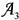is the set formed by the two subsets e3 (explicit category attributes) and i3 (implicit category attributes), each of them obtained by the union of e1 with e2 and of i1 with i2, respectively;

s3 is a union of the sets of the summary values of s1 and s2. Notice that these values, sometimes, have to be recomputed, for example, if the summary type is "percentage" and the grand total is normalized to 100. This recomputation is transparent to the user.

For this operator the term concatenation has also been used. This operator (with this name), was proposed in Ozsoyoglu, Ozsoyoglu, & Mata (1985), and carries out a column category attribute forest of ordered sets which is the concatenation of the two MAD (called summary tables in that paper) in input and an ordered cell attribute set (concatenation of the two cell attribute sets of the previous column category attribute forests) so that, if this cell is in the first MAD, then its cell attribute is in the first ordered cell attribute set; otherwise, in the second ordered cell attribute set, this applies to each cell in the MAD.

Finally, another term used for this operator is juxtapoint. It refers to two different situations. The former regards MAD with a different summary type of the measure. In this case the result of the operation consists of a new composite MAD, and the juxtaposition happens along one dimension common to the two MADs. The latter consists of a new MAD for which, if the summary attribute of the original MAD is not summable (e.g., average), the instances inside its cells have to be recomputed, according to the aggregation function applied to the microdata to obtain it.Multidimensional Databases: Problems and Solutions
ISBN: 1591400538
EAN: 2147483647
Year: 2003
Pages: 150